# 射频 - 天线基础知识

## 天线的原理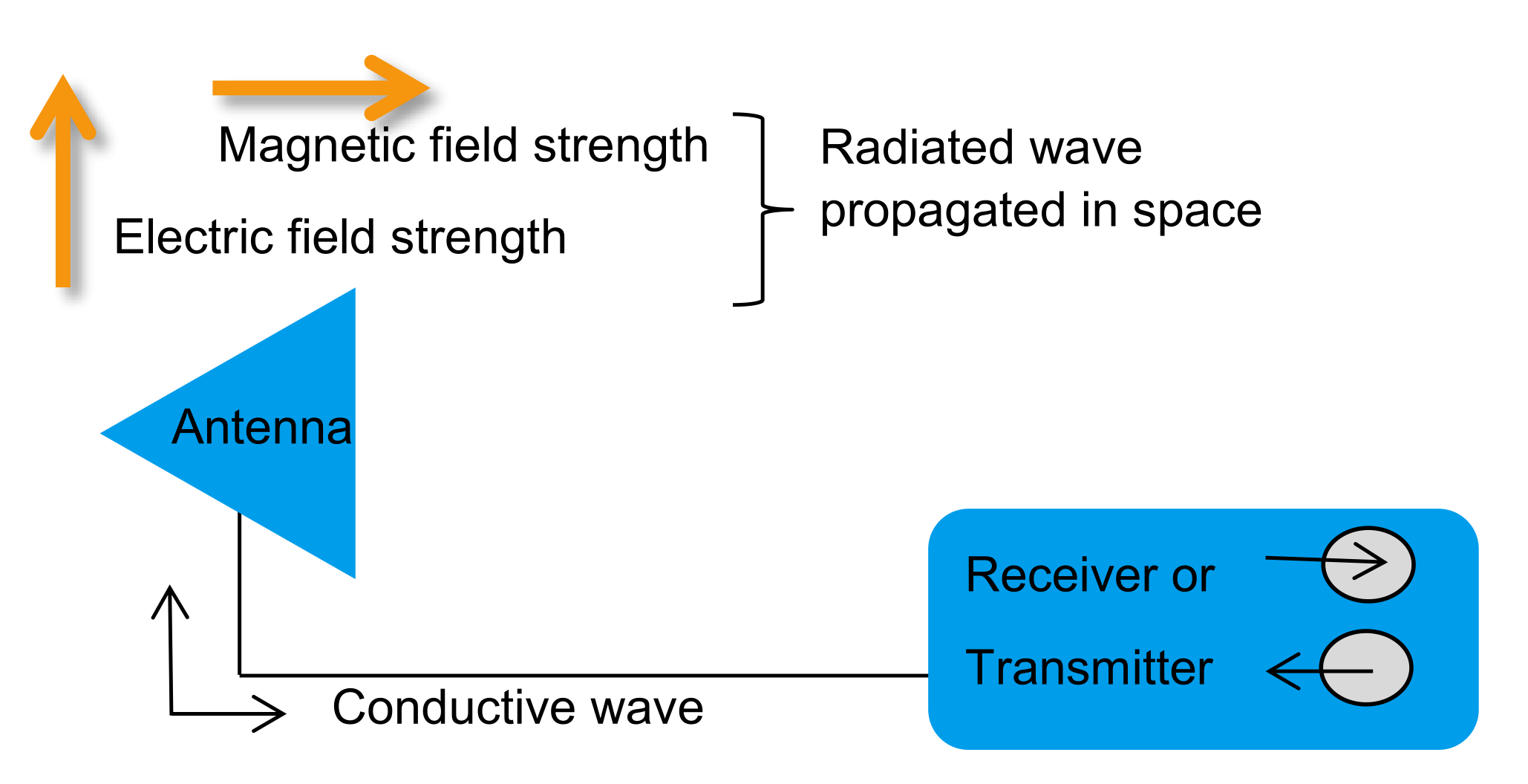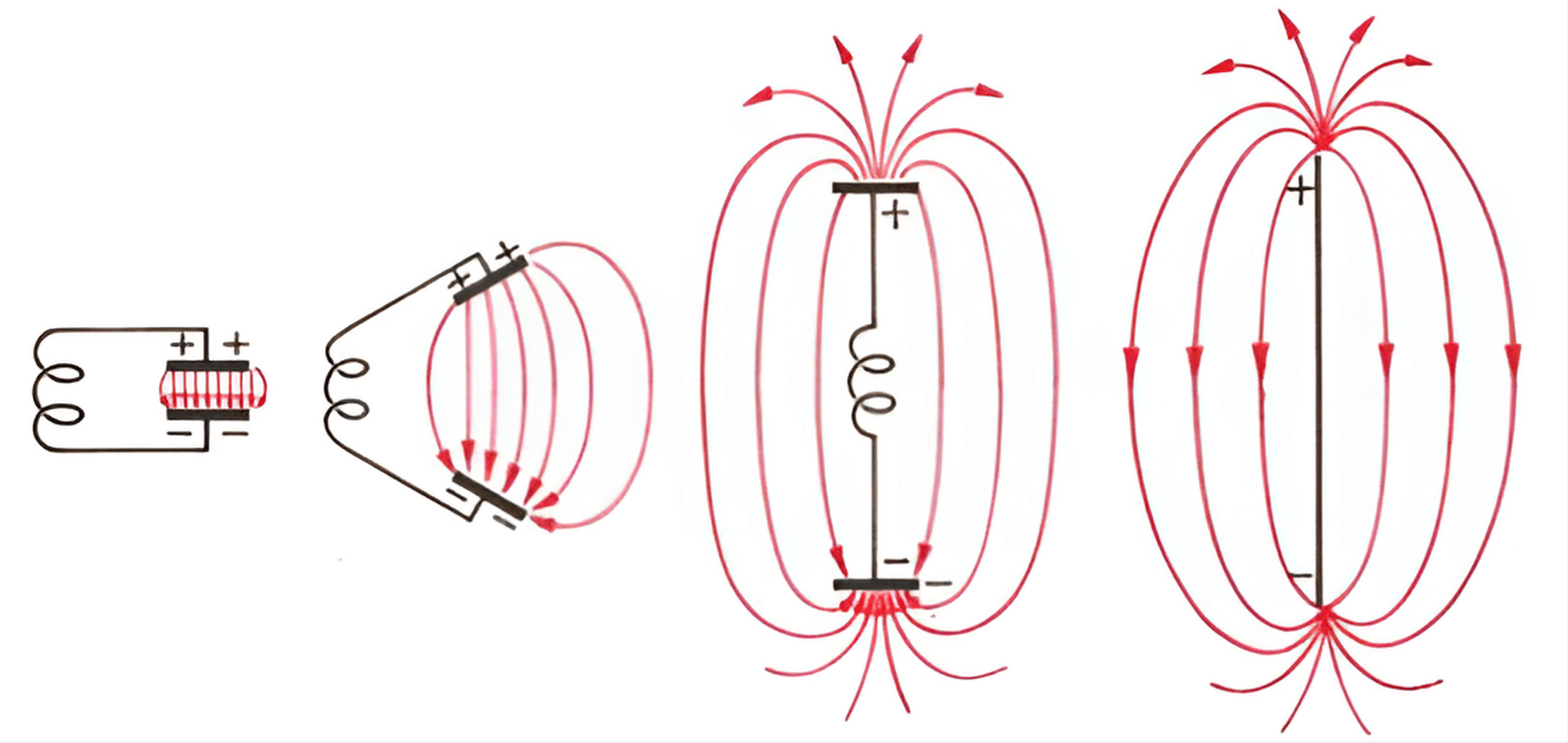## 天线的参数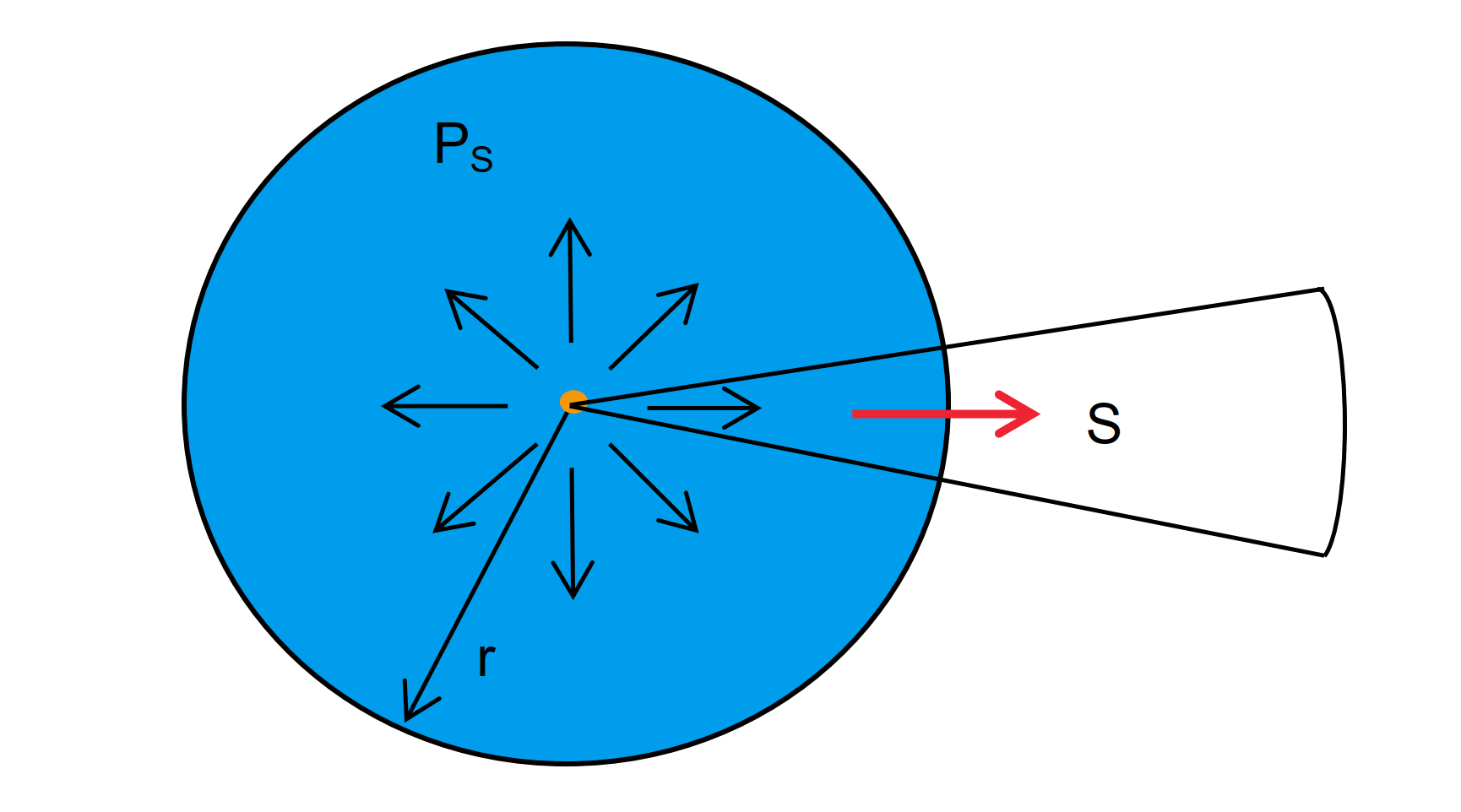$S=\frac{P_S}{4\pi r^2}$

$S=E \cdot H$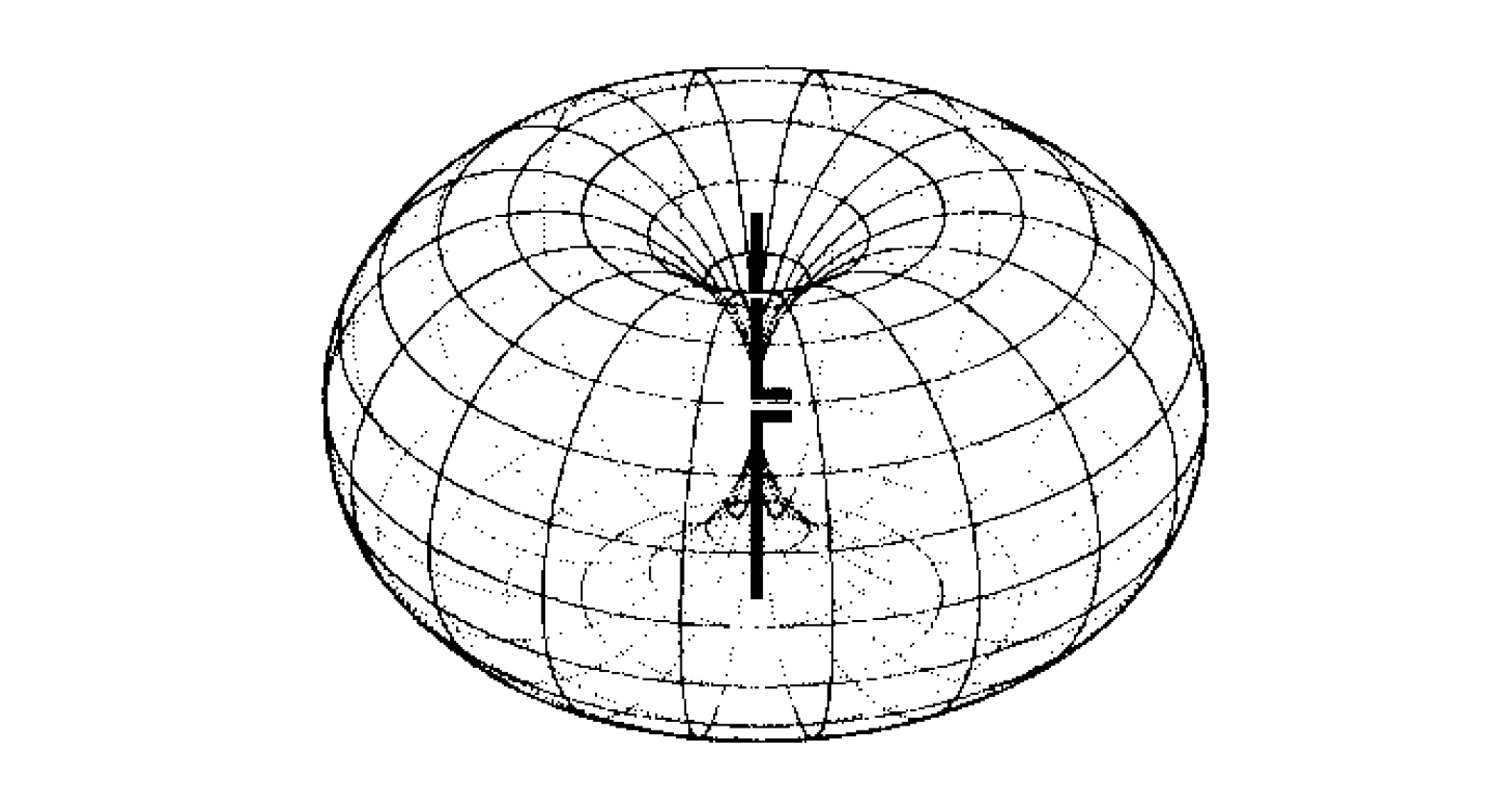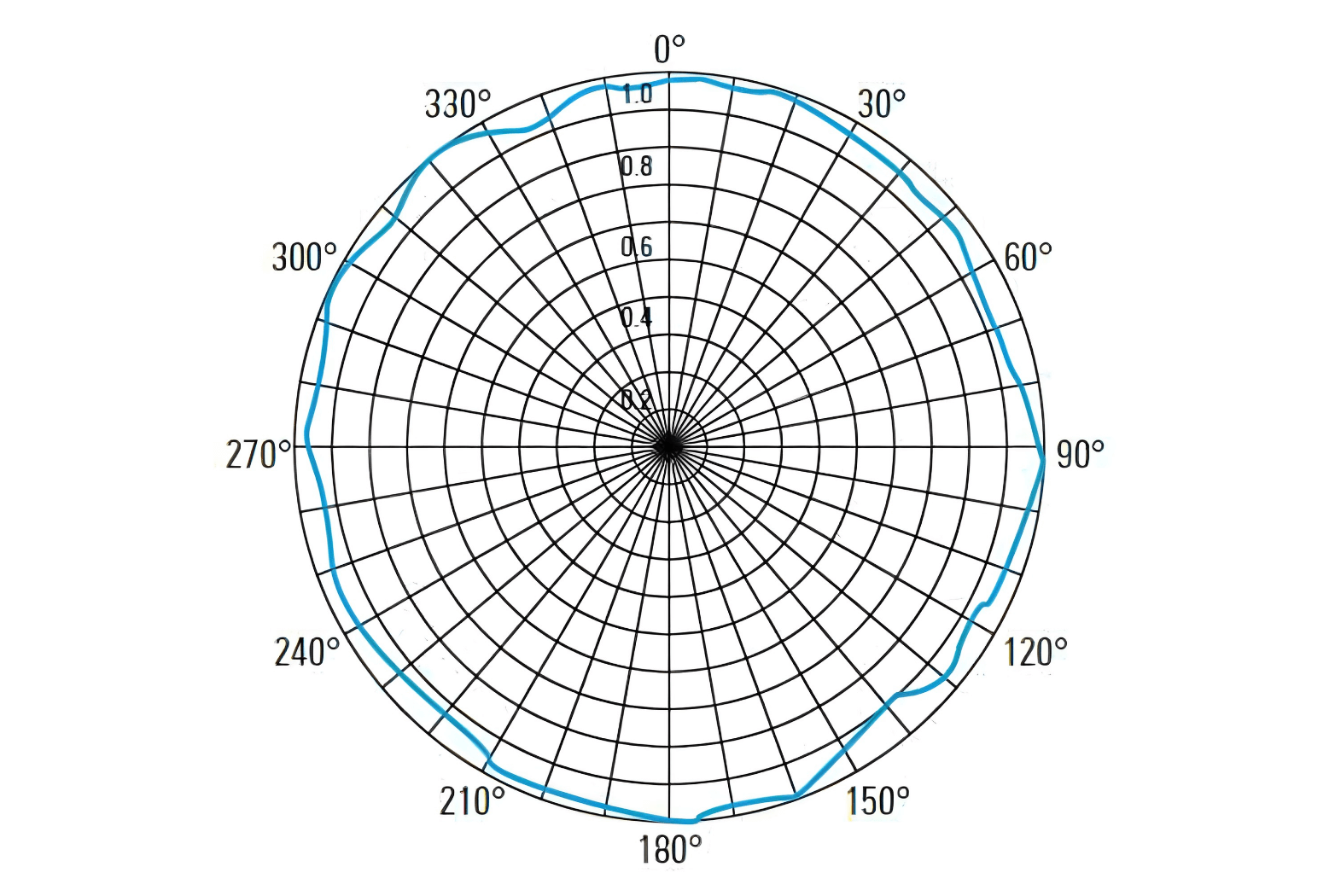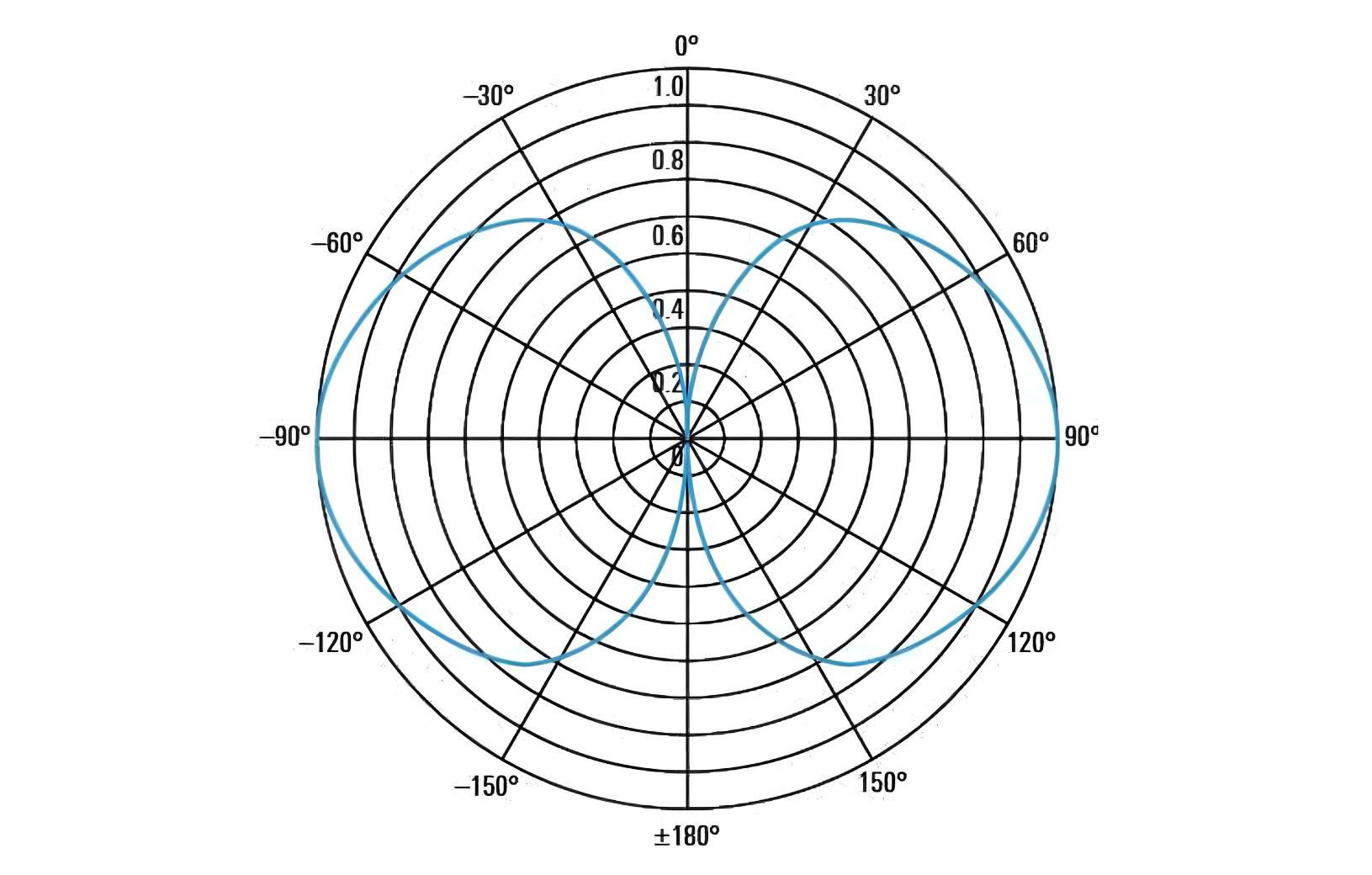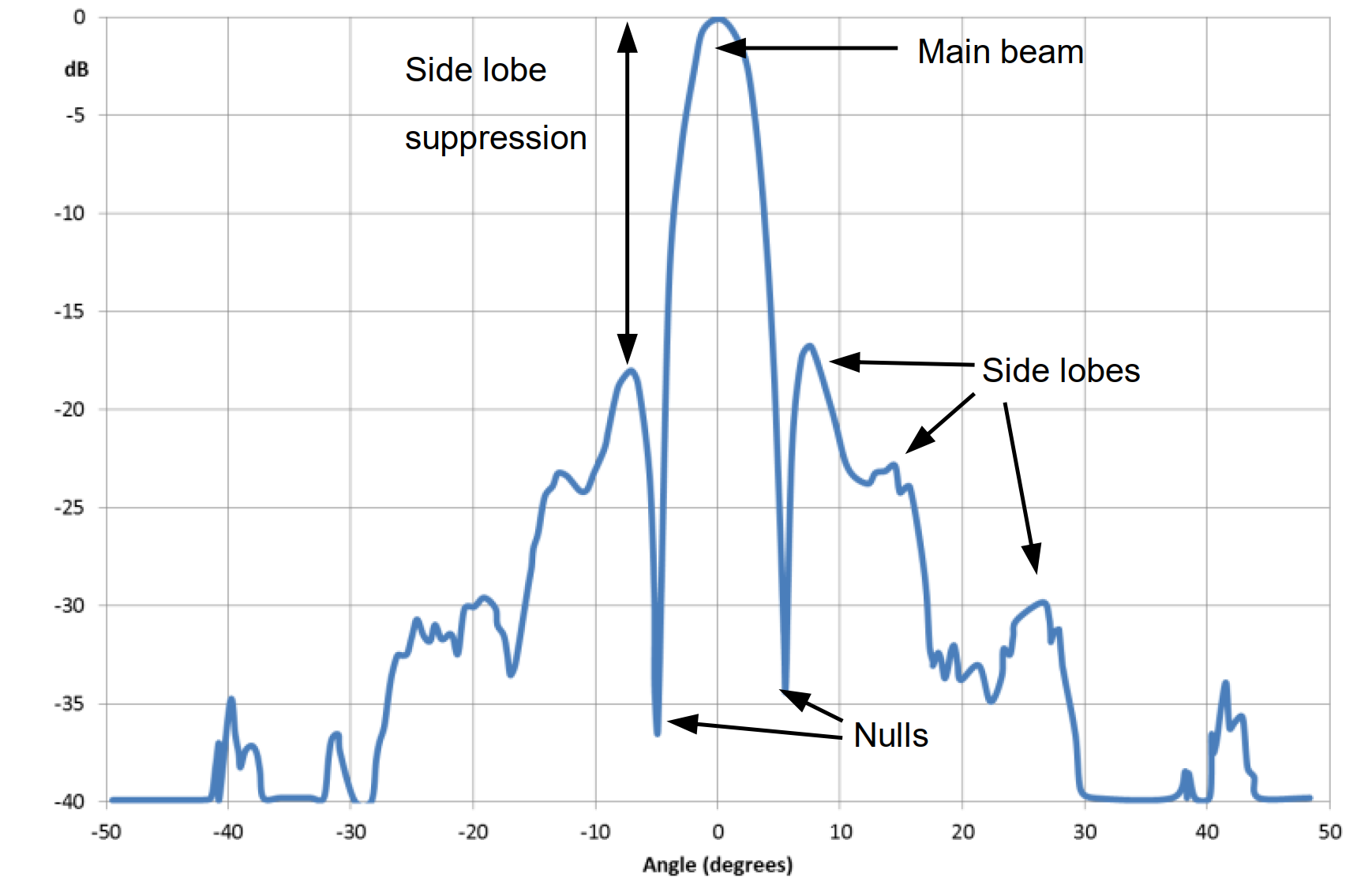• 旁瓣抑制（Side Lobe Suppression，或旁瓣电平）：表示主瓣与最高旁瓣之间的差值。
• 水平半功率波束宽度（Half-Power Beamwidth, HPBW）：表示从主瓣最大高度下降 3dB 的左右两个角度之间的范围，通常在水平和垂直两个 2D 辐射方向图中呈现。
• 前后功率比（Front-to-Back Ratio）：表示定向天线正向峰值增益与背面（180°）增益的比值，通常用 dB 表示。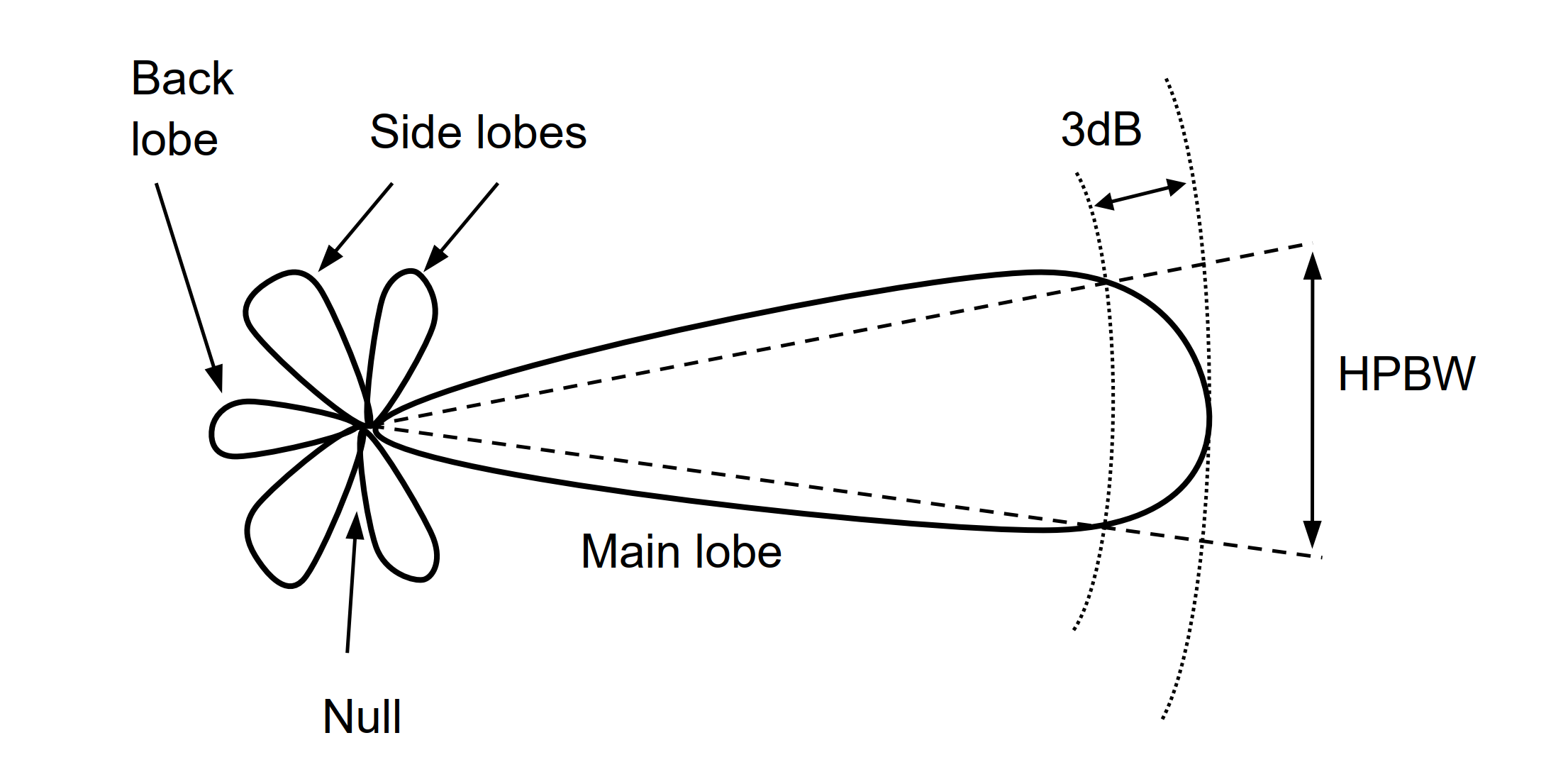### 方向性（Directivity）

$\vec S=\vec E \times \vec H$

$D=\frac{F_{max}}{F_i}$

### 效率（Efficiency）

$\varepsilon_R=\frac{P_{refl}}{P_{forw}}\cdot 100\%$

$\varepsilon_r=M_L\cdot\varepsilon_R$

### 增益（Gain）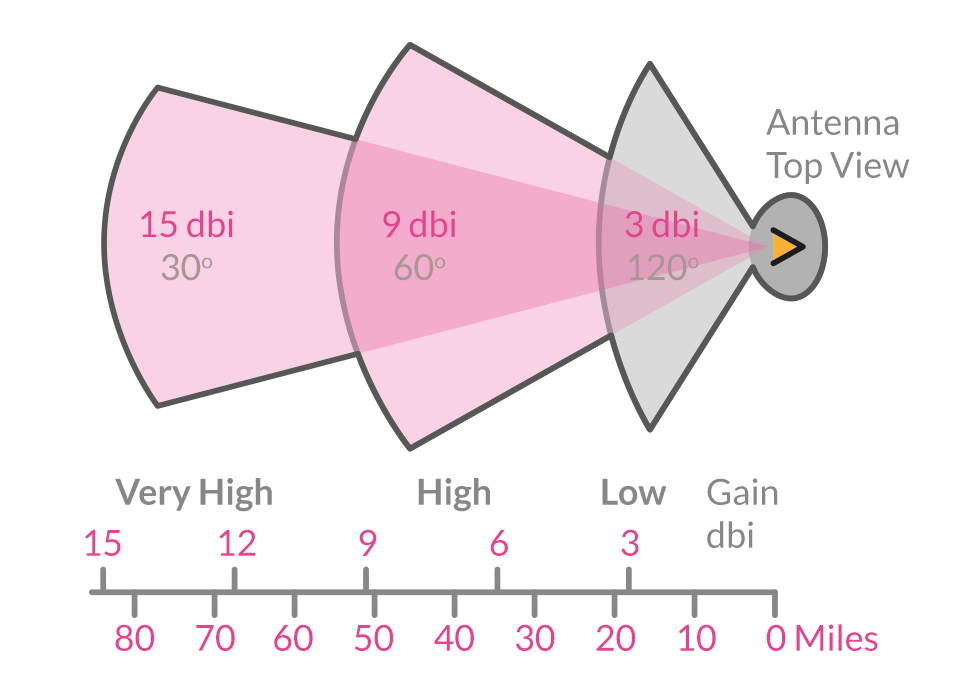$G=\frac{F_{max}}{F_{i0}}$

$G=\eta \cdot D$

• 天线是无源器件，不产生能量。天线增益只是一种将能量有效集中向某特定方向辐射 / 接受电磁波的能力。
• 天线的增益由振子叠加而产生。增益越高，天线长度越长。增益每增加 3dB，体积就就会增大一倍。

### 实际增益（Practical Gain）

$G_{pract}=(1-|r|^2)\cdot G$

### 有效面积（Effective Area）

$A_W=\frac{P{rmax}}{S}$

$A_W=A_g\cdot q$

$A_W=\frac{\lambda ^2}{4\pi}\cdot G$

### 输入阻抗（Input Impedance）

$Z_{in}=R_{in}+jX_{in}$

$R_{in}=R_R+R_L$

### 阻抗匹配（Impedance Matching）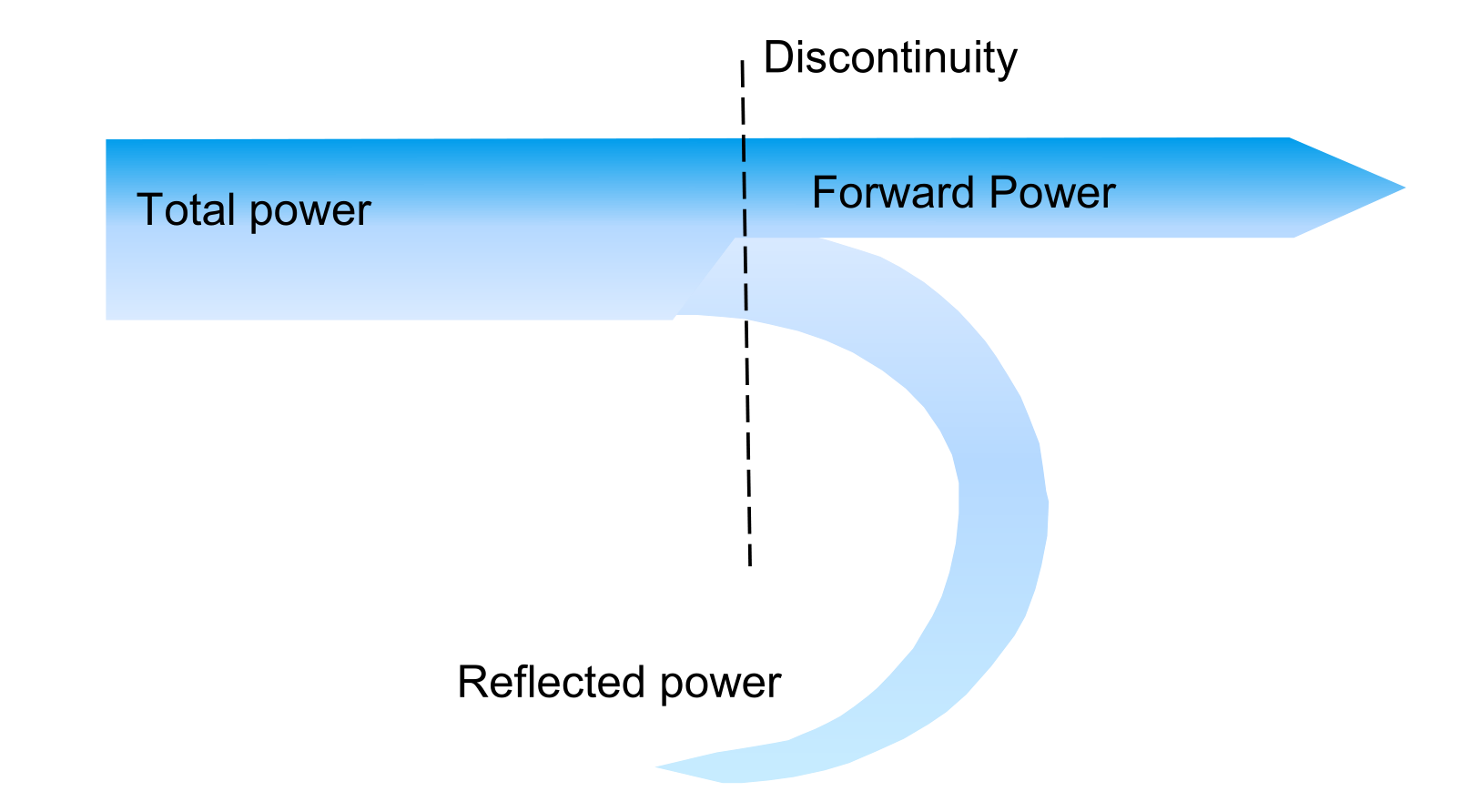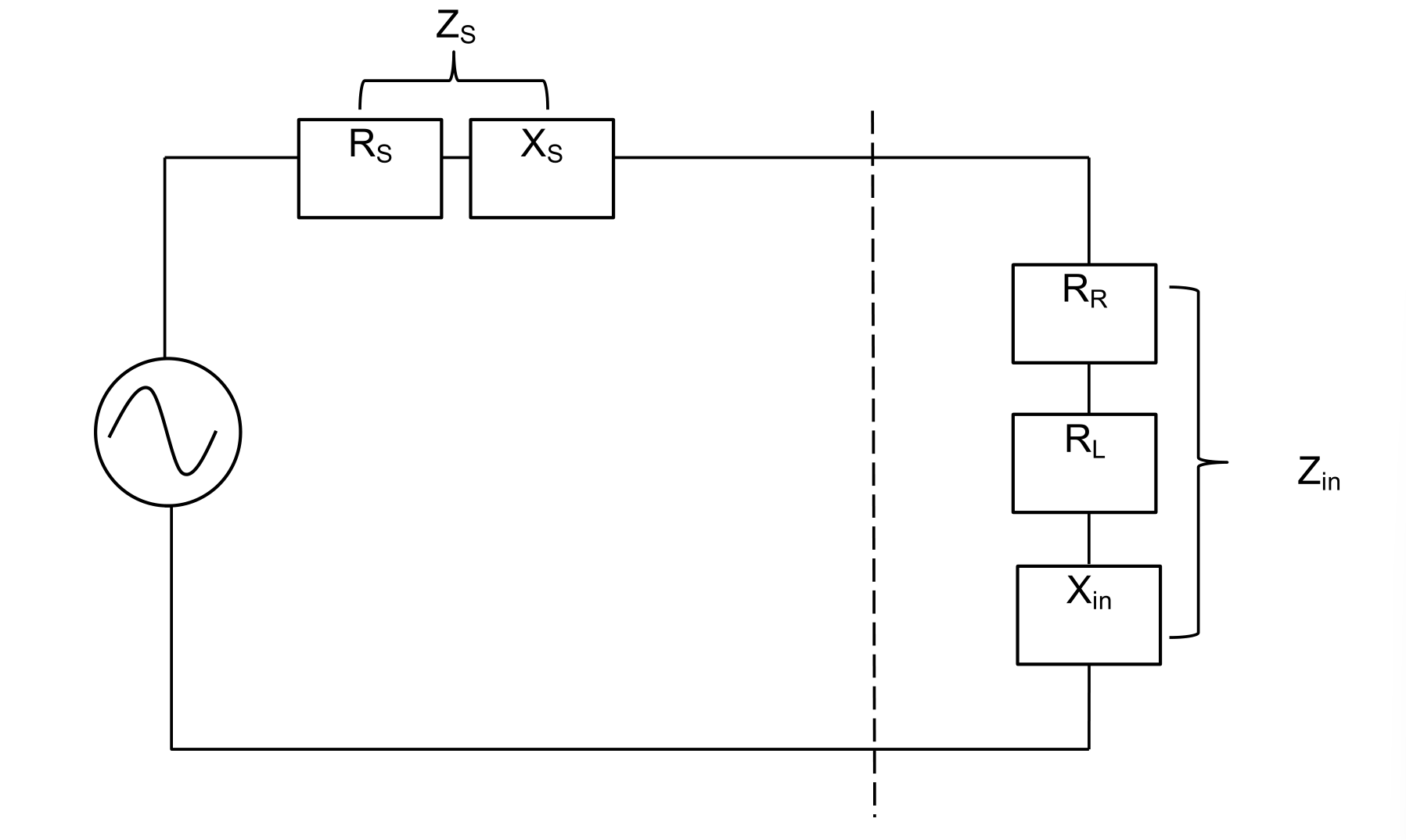### 驻波比（Voltage Standing Wave Ratio, VSWR）

$s=\frac{V_{max}}{V_{min}}=\frac{I_{max}}{I_{min}}$

$s=\frac{|V_{forw}|+|V_{refl}|}{|V_{forw}|-|V_{refl}|}=\frac{\sqrt{P_{forw}}+\sqrt{P_{forw}}}{\sqrt{P_{forw}}-\sqrt{P_{forw}}}$

$r=\frac{V_{refl}}{V_{forw}}$

$r=\frac{1+|r|}{1-|r|}$

$a_r=-20log|r|$
$=-20log\frac{V_{refl}}{V_{forw}}=-10log\frac{P_{refl}}{P_{forw}}$

VSWR R $$a_r$$ 反射的能量
1.002 0.001 60dB \
1.01 0.005 46dB \
1.1 0.05 26dB 0.2%
1.2 0.1 20dB 0.8%
1.5 0.2 14dB 4%
2.0 0.33 9.5dB 11.1%
2.0 0.5 6dB 25%
5.0 0.67 3.5dB 44.4%

### 天线因子（Antenna Factor）

$K=\frac{E}{V}$

$k=20log K$

$K=\frac{9.73}{\lambda \cdot \sqrt{G}}$
$k=-29dB+20log(\frac{f}{MHz})-g$

### 带宽（Bandwidth）

$BW=\frac{f_H}{f_L}$

$BW=\frac{f_H-f_L}{f_C}\cdot100\%$